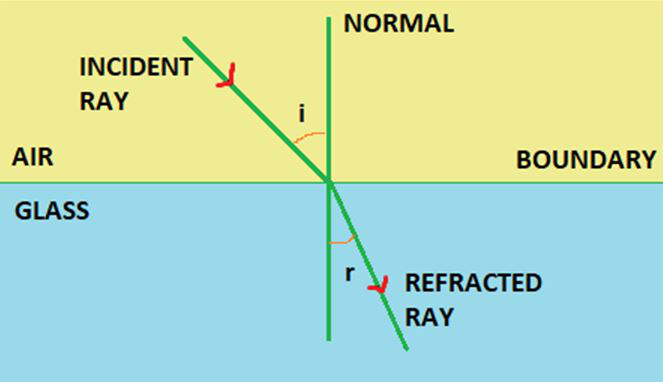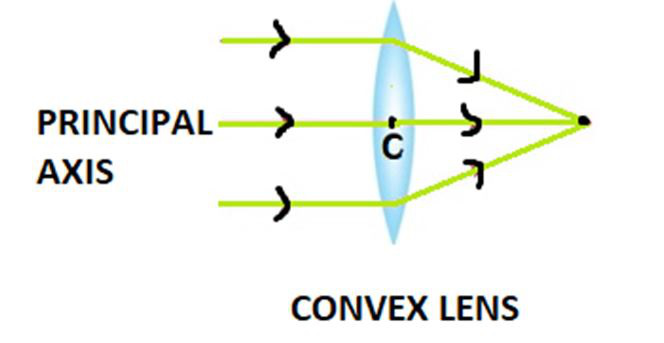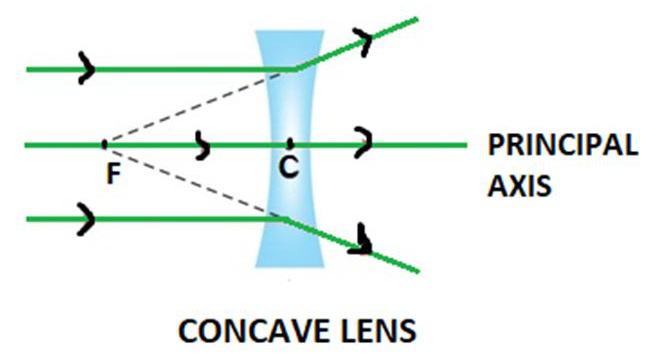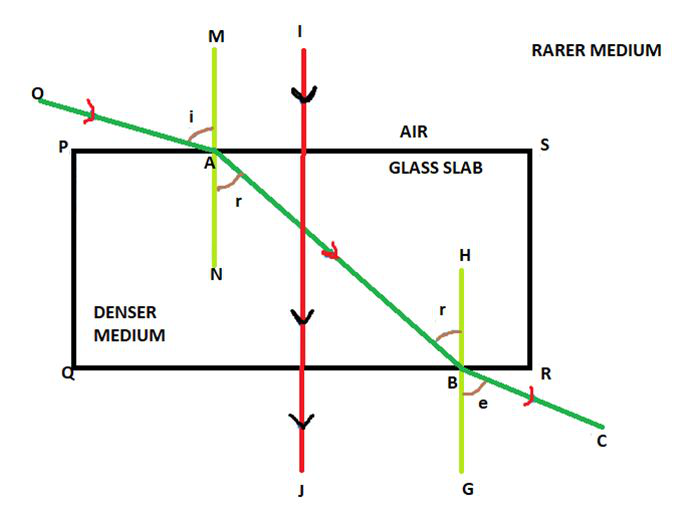# Refraction of Light

• Last Updated : 18 Mar, 2021

By seeing the common optical phenomena that surround us, we may conclude that the light seems to travel in straight lines. Sometime in the 20th century, the wave theory of light often became slightly inadequate for cases of the interaction of light with matter, and light often behaves somewhat like a stream or system of particles. This discussion about the true nature of light continued for years till a modern quantum theory of light came in which light is neither a become slightly wave nor a ‘particle’ in nature – the new theory relates the particle properties of light with the wave nature of it. The refraction of light is explained briefly using the straight-line propagation of light in this article.

### Refraction of Light

It sometimes appears that when light is travelling obliquely from one medium to another medium, the direction of the propagation of light in the second medium somehow changes. This certain phenomenon is known as what we call the refraction of light. In simple words, it describes the change in the velocity or speed of light when it travels from one medium to another medium. The refraction of light depends upon the velocity of the medium we use and the nature of another medium from which the light comes. There are certain laws also which this refraction phenomenon follows.

### Causes of Refraction

Change of Speed changes the Direction of light that travels through the medium:

The light ray undergoes refraction at the surface whenever it travels at an angle into a medium of slightly different refractive index. The change in the speed of light results in a change in the direction of light ray. For instance, consider air travelling into the water. The speed of light further decreases as it continues to travel at a certain different angle.Refraction of light in glass is described in the figure given above. When a light ray travels from air into the glass, the light slows down gradually and changes its direction slightly. When the light ray travels from a less dense substance to a denser substance, the refracted light bends or deviates more towards the normal imaginary line. If the light wave falls on the boundary in a direction that is perpendicular or at right angles to it, the light ray doesn’t suffer refraction in spite of the change in its speed.

### Laws of Refraction

The refraction of light travelling through different mediums follows some laws. There are two laws of refraction as stated below which at the sight of refraction, the light follows, and we see the refracted image of the object.

• The reflected, incident and the normal at the point of incidence all will tend to lie in the same plane.
• Secondly, the ratio of sin of angle of the incidence and refraction is constant which is termed as Snell’s law.=constant

Where i= angle of incidence, r= angle of refraction, the constant value depends on the refractive indexes of the two mediums. It is their ratio.

### Refraction in Real Life

• Mirage in deserts and looming are types of optical illusions in our eyes that are a result of the refraction of light.
• A swimming pool always seems or looks much shallower than it really is because of the light that comes from the bottom of the pool bends at the surfaces due to the refraction of light.
• The formation of a rainbow by water drops is an example of refraction as the sun rays bend through the raindrops or water drops in the air resulting in the rainbow.
• When white light passes through the prism it gets split into its component seven colors(VIBGYOR) — red, orange, yellow, green, blue and violet due to the refraction of light.

### Applications of Refraction of Light

Refraction has many wide and common applications in optics and also in technology. A few of them are given below:

• A lens uses refraction phenomenon to form an image of an object or body for various purposes, such as for magnification.
• Spectacles that are worn by people with defective vision use the principle of refraction.
• Refraction is used in peepholes of the house doors for safety, in cameras, inside movie projectors and also in telescopes.

### Refractive Index

Refractive index also called the index of refraction enables us to know how fast light travels through the material medium.

Refractive Index is a dimensionless quantity. For a given material or medium, the refractive index is considered the ratio between the speed of light in a vacuum (c) to the speed of light in the medium (v) on which it goes. The Refractive index for a medium is represented by small n, and it is given by the following formula:Where c = speed of the light in vacuum, v = speed of light in the medium.

The given velocities of light in different media can give the refractive index by the following also where the first medium is not vacuum:The n21 = refractive index of 2 with respect to 1.

Based on the given refractive index of the material or medium, the light ray either changes its direction, or it bends at the junction which separates the two given media. If the light ray that travels from a certain medium to another of a slightly higher refractive index, it bends towards the normal in that case when travelling from rarer to a denser medium, or else it bends away from the normal when traveling from denser to rarer medium.

The following table gives an idea about the different refractive index of mediums. The one with a higher refractive index is optically denser than a material with a low refractive index which becomes a rarer medium. The values are calculated against vacuum or air, and so they are the absolute refractive indexes.

### Refraction through Lenses

Light rays after passing from air to glass are refracted. By utilizing special types of spherical lenses, which are mainly concave or convex, the light rays passing from air to the lens in turn, are either diverged from the surface or converged from the surface of the lens. Lenses are used in telescopes and microscopes. They are also required in cameras and for projectors. Our own eyes have a convex lens and so are an example of a spherical lens. At first, the image is inverted, but when the optical nerves in the blind spot transfer the impulses to the brain it processes it, and we perceive the image in its correct orientation. Important terms related to refraction through lenses:

1. Centre of Curvature: The centre of the actual glass sphere, of which the given lens forms a part.
2. Principal Axis: When two spheres are part of a given lens, it is the imaginary line that joins the centre of curvatures of both spheres.
3. Principal Focus: It is a point located on the principal axis, where the light rays which are parallel to the principal axis meet in case of a convex lens (or appear to meet after we do extrapolation in of a concave lens).
4. Optical Centre: It is a point located within the lens or at the centre where the diameter of the lens and the principal axis meet together.
5. Focal Length: The distance between the focus and the optical centre

### Convex Lens

A convex lens is also called a converging lens since it converges the light rays that fall on it. The lens surface is somewhat convex in nature. If a ray of light passes parallel to the principal axis of a convex lens coming from an infinite object, the refracted ray passes through the focus and this point is called the principal focus of the convex lens. If the incident ray passes through the optical centre of the given lens, the refracted ray from the lens does not deviate at all. If the incident ray if passes through the focus of it, the refracted ray then after refraction passes parallel to the principal axis.Principal Focus of Convex Lens

### Concave Lens

A concave lens is also called or described as a diverging lens since it diverges the light rays that are incident on it. The lens surface is somewhat concave in nature. An incident light ray that comes parallel to the principal axis of a concave lens passes through it and diverges. When extrapolated backwards, it appears to pass through the focus this point is referred to as principal focus. If the incident light ray passes through the optical centre of the lens, like in the convex lens, the refracted ray passes through it without any deviation from it. If the incident light ray after refraction through the lens passes through the focus of the lens, it is refracted parallel to the principal axis.Principal of Concave Lens

### Refraction through Glass Slab

As we can observe below, the refraction through a glass slab takes place at the two parallel and equal and opposite surfaces. As we can see the incident ray first undergoes refraction at the PS surface and the refracted ray bends towards the normal and again it is incident on the other surface which is equal to the first one and that is QR. Through a glass slab, the light before coming back to the air suffers refraction two times. At the second time, the refracted ray bends away from the normal. If the light is incident at right angles then it will pass through the glass slab without any deviation.Here, i = incident angle, r = refracted angle, e = emergent angle and OA = incident ray, BC = emergent ray, AB = refracted ray, MN = normal, HG = normal.

### Sample Problems

Question 1: What is the constant value if the angle of incidence is 22° and the angle of refraction is given to be15°?

Solution:

As we know,= constant

Given sin i = sin 22° and sin r = sin 22°

Putting the values of angles from log table we getHence, the constant is 1.44.

Question 2: What is the constant value if the angle of incidence is 30° and the angle of refraction is given to be 46°?

Solution:

As we know,

{sin i} / {sin r} =constant

Given sin i= sin 30° and sin r= sin 46°

Putting the values of angles from log table we getHence, the constant is 1.44.

Question 3: What is the value of the sin of the angle of incidence if the angle of refraction is given to be sin 35°? The constant is mentioned as 1.33

Solution:

As we know,

{sin i}/{sin r} =constant

Given constant= 1.33 and sin r = sin 35° = 0.57

Putting the values of angles from log table we get{sin i} = 1.33 × 0.57

{sin i} = 0.75

Hence, sin i= 0.75

Question 4: Define the term Refraction.

The change that occurs in the direction of a wave when light passes from one medium to the other is known as refraction of light.

Question 5: When is the refraction of light not possible?

When the light is incident perpendicular to the boundary or surface, refraction of light is not possible.

Question 6: Calculate the speed of light in diamond with respect to air. Take the absolute refractive index of glass from the table.

Solution:

As we know we can calculate refractive index by the following formula,Where refractive index of diamond n= 2.42, c = 3 × 108 m/sHence, the velocity or speed of light in glass is vd = 1.24 × 108 m/s

Question 7: Calculate the speed of light in glass with respect to air. Take the absolute refractive index of glass from the table.

Solution:

As we know we can calculate refractive index by the following formula,Where refractive index of glass n= 1.52, c= 3 × 108 m/sHence, the velocity or speed of light in glass is vg = 1.97 × 108 m/s

Question 8: Calculate the speed of light in water with respect to air. Take the absolute refractive index of glass from the table.

Solution:

As we know we can calculate refractive index by the following formula,Where refractive index of water n= 1.53, c = 3 × 108 m/sHence, the velocity or speed of light in water is vw = 1.96 × 108 m/s

My Personal Notes arrow_drop_up